Home | | Chemistry 11th std | Brief questions and answers: Fundamentals of Organic Chemistry

# Brief questions and answers: Fundamentals of Organic Chemistry

Brief questions and answers, Answer the following questions, Numerical Problems Questions with Answers, Solution : Chemistry: Fundamentals of Organic Chemistry

Fundamentals of Organic Chemistry

31. Give the general characteristics of organic compounds?

● They are convalent compounds of carbon.

● They are insoluble in water and readily soluble in organic solvent such as benzene, toluene, ether, chloroform etc.......

● Many of the organic compounds are inflammable (except CCl4)

● They possess low boiling and melting points due to their convalent nature.

● Organic compounds are characterised by functional groups. A functional group is an atom or a specific combination of bonded atoms that react in a characteristic way, irrespective of the organic molecule in which it is present.

● They exhibit Isomerism.

● They are characterised by homologous series.

32. Describe the classification of organic compounds based on their structure.33. Write a note on homologous series.

Homologous series : A series of organic compounds each containing a characteristic functional group and the successive members differ from each other in moleculr formula by a CH2 group is called homologous series. Eg Alkanes : Methane (CH4), Ethane (C2H6), Propane (C3H8) etc...

Alcohols : Methanol (CH3OH), Ethanol (C2H5OH), Propanol (C3H7OH) etc...

● Compounds of the homologous series are represented by a general formula Alkanes CnH2n+ 2 Alkenes CnH2n , Alkynes CnH2n- 2 and can be prepared by general methods.

● They show regular graduation in physical properties but have almost similar chemical property.

34. What is meant by a functional group? Identify the functional group in the following compounds.

(a) acetaldehyde

(b) oxalic acid

(c) di methyl ether

(d) methylamine

a) acetaldehyde

b) oxalic acid

c) di methyl ether

d) methylamine

A functional group is an atom or a specific combination of bonded atoms that react in a characteristic way irrespective of the organic molecule in which it is present.35. Give the general formula for the following classes of organic compounds

a) Aliphatic monohydric alcohol

b) Aliphatic ketones.

c) Aliphatic amines.

a) Aliphatic monohydric alcohol: R-OH

b) Aliphatic ketones:c) Aliphatic amines: R - NH2

36. Write the molecular formula of the first six members of homologous series of nitro alkanes.

1. CH3NO2

2. CH3-CH2-NO2

3. CH3 - CH2 - CH2 - NO2

4.5. CH3 - CH2 - CH2 - CH2 NO2

6.37. Write the molecular and possible structural formula of the first four members of homologous series of carboxylic acids.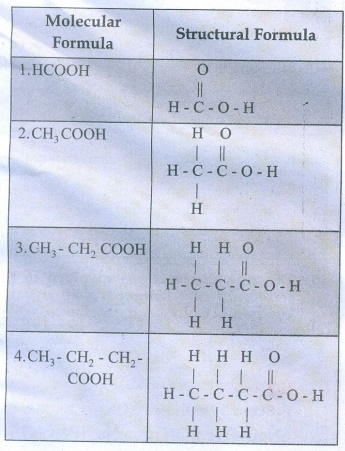38. Give the IUPAC names of the following compounds.Solution:

(i) 2,3,5-trimethylhexane

(ii) 2-bromo-3-methylbutane

(iii) methoxymethane

(iv) 2-hydroxybutanal

(v) buta-1,3-diene

(vi) 4-chloropent-2-yne

(vii) 1-bromobut-2-ene

(viii) 5-oxohexanoic acid

(ix) 3-ethyl-4-ethenylheptane

(x) 2,44-trimethylpent-2-ene

(xi) 2- methyl-1-phenylpropan-1-amine

(xii) 2,2- dimethyl-4oxopentanenitrile

(xiii) 2-ethoxypropane

(xiv) 1-fluoro-4-methyl-2-nitrobenzene

(xv) 3-bromo-2-methylpentanal

(xvi) Acetophenone

39. Give the structure for the following compound.

i.    3- ethyl - 2 methyl -1-pentene

ii.   1,3,5- Trimethyl cyclohex - 1 -ene

iii.  tertiary butyl iodide

iv.  3 - Chlorobutanal

v.   3 - Chlorobutanol

vi.  2 - Chloro - 2- methyl propane

vii. 2,2-dimethyl-1-chloropropane

viii.    3 - methylbut -1- ene

ix.  Butan - 2, 2 - diol

x.   Octane - 1,3- diene

xi.  1,5- Dimethylcyclohexane

xii. 2-Chlorobut - 3 - ene

xiii.    2 - methylbutan - 3 - ol

xiv.    acetaldehyde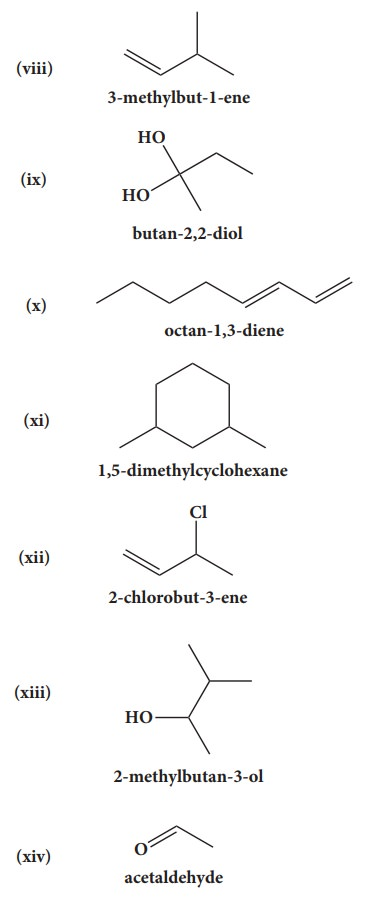40. Describe the reactions involved in the detection of nitrogen in an organic compound by Lassaigne method.

Na + C + N → NaCN

(from organic compounds)

FeSO4 + 2 NaOH → Fe(OH)2 + Na2SO4

6NaCN + [Fe(OH)2] → Na4 [Fe(CN)6] sod. ferro cyanide + 2NaOH

3Na4[Fe(CN)6] + FeCl3 → Fe4 [Fe(CN)6]3 + 12NaCl

Prussian blue (or) green ppt. Ferric ferro cyanide

41. Give the principle involved in the estimation of halogen in an organic compound by carius method.

Estimation of halogens: carius method:

A known mass of the organic compound is heated with fuming HNO3 and AgNO3. C, H & S get oxidized to CO2, H2O & SO2 and halogen combines with AgNO3 to form a precipitate of silver halide.The ppt of AgX is filtered, washed, dried and weighed. From the mass of AgX and the mass of the organic compound taken, percentage of halogens are calculated.

42. Give a brief description of the principles of i) Fractional distillation ii) Column Chromatography

i) Fractional distillation:

● This is one method to purify and separate liquids present in the mixture having their boiling point close to each other.

● In the fractional distillation, a fractionating column is fitted with distillation flask and a condenser.

● A thermometer is fitted in the fractionating column near the mouth of the condenser.

● This will enable to record the temperature of vapour passing over the condenser.

● The process of separation of the components in a liquid mixture at their respective boiling points in the form of vapours and the subsequent condensation of those vapours is called fractional distillation.

● The process of fractional distillation is repeated, if necessary.

● This method finds a remarkable application in distillation of petroleum, coal-tar and crude oil.

ii) Column chromatography:

● This is the simplest chromatographic method carried out in long glass cloumn having a stop cock near the lower end.

● This method involves separation of a mixture over a column of adsorbent (Stationery phase) packed.

● In the column a plug of cotton or glass wool is placed at the lower end of the column to support the adsorbent powder.

● The tube is uniformly packed with suitable absorbent constitute the stationary phase. (Activatied aluminum oxides (alumina), Magnesium oxide, starch are also used as absorbents).

● The mixture to be separated is placed on the top of the adsorbent column. Eluent which is a liquid or a mixture of liquids is allowed to flow down the column slowly. Different components depending upon the degress to which the componenets are adsorbed and complete separation takes place.

● The most readily adsorbed substances are retained near the top and others come down to various distances in the column.

43. Explain paper chromatography (or) Partition chromatography.

● Paper chromatography (PC) is an example of partition chromatography.

● A strip of paper acts as an adsobent. This method involves continoues differential portioning of components of a mixture between stationary and mobile phase.

● In paper chromatography, a special quality paper known as chromatography paper is used. This paper act as a stationary phase.

● A strip of chromatographic paper spotted at the base with the solution of the mixture is suspended in a suitable solvent which act as the mobile phase.

● The solvent rises up and flows over the spot. The paper selectively retains different components according to their different partition in the two phases where a chromatogram is developed.

● The spots of the separated colored compounds are visible at different heights from the position of initial spots on the chromatogram.

● The spots of the separated colorless compounds may be observed either under ultraviolent light or by the use of an appropriate spray reagent.

44. Explain varions types of constitutional isomerism (structural isomerism) in organic compounds

Constitutional Isomers (Structural Isomerism):

This type of Isomers have same molecular formula but differ in their bonding sequence.

Structural Isomerism

|→ a) Chain or nuclear or skeletal isomerism

|→ b) Position Isomerism

|→ c) Functional Isomerism

|→ d) Metamerism

|→ e) Tautomerism

a) Chain Isomerism:

Isomers have similar molecular formula but differ in the nature of carbon skeleton (ie straight or branched)

Eg : CH3-CH2-CH2-CH2-CH3

n - pentaneb) Position Isomerism:

Isomers with the same molecular formula and carbon skeleton but differ in the position of substituent or functional group or an unsaturated linkage.

Eg. M.FC5H10

CH3- CH2- CH2 - CH = CH2

Pent −1 - ene

CH3-CH2-CH = CH-CH3

Pent-2-ene

c) Functional Isomerism:

Different compounds with the same molecular formula but different functional groups are said to exhibit functional isomerism.d) Metamerism : Isomerism arising due to unequal distribution of carbon atoms on either side of the functional group or different alkyl groups attached to the either side of the same functional group and having same molecular formula.

Eg C4H10O

CH3- O - C3H7

methyl propyl ether

C2H5-O - C2H5

Diethyl ether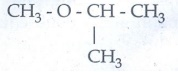methyl isopropyl ether

e) Tautomerism : Single compound exists in two readily interconvertible structures that differ in the relative position of atleast one atomic nucleus (hydrogen)

Eg:

H - C ≡ N ↔ H – N ≡ C

Hydrogen cyanide  ↔ Hydrogen isocyanide45. Describe optical isomerism with suitable example.

1) Compound having same physical and chemical property but differ only in the rotation of plane of the polarized light are known as optical isomer and the phenomenon is known as optical isomerism.

2) Some organic compounds such as glucose have the ability to rotate the plane polarized light and they are said to be optically active compounds and this property of a compound is called optical activity.

3) The optical isomer, which rotates the plane of the plane polarised light to the right or in clock wise direction is said to be dextrorotatary (dexter means right) denoted by the sign (+).

4) Where as the compound which rotates to the left or anticlock wise is said to be leavo rotatory (leavues means left) denoted by sign(-).

Eg;46. Briefly explain geometrical isomerism in alkene by considering 2- butene as an example.

● Geometrical isomers which have different arrangement of groups or atoms around a c=c. This isomeris occur due to restricted rotation of double bonds, or about single bonds in cyclic compounds.● The cis isomer is one in which two similar groups are on the same side of the double bond.

● The trans isomer is that in which the two similar groups are on the opposite side of the double bond.

47. 0.30 g of a substance gives 0.88 g of carbon dioxide and 0.54 g of water calculate the percentage of carbon and hydrogen in it.

Weight of organic substance (w) = 0.30g

Weight of water (x) = 0.54g

Weight of CO2(y) = 0.88g

Percentage of carbon = (12/44) × (y/w) × 100

= (12/44) × (0.88/0.30) × 100

= 80%

Percentage of Hydrogen = (12/18) × (x/w) × 100

= (2/18) × (0.54/0.30) × 100

= 20%

48. The ammonia evolved form 0.20 g of an organic compound by kjeldahl method neutralised 15ml of N/20 sulphare acid solution. Calculate the percentage of Nitrogen.

Percentage of nitrogen = [ 1.4 × normality of acid × volume of acid used ] / [ Mass of the compound taken ]

= [ 1.4 × 0.05 × 15ml ] / 0.20

= 5.25%

49. 0.32 g of an organic compound, after heating with fuming nitric acid and barium nitrate crystals is a sealed tube game 0.466 g of barium sulphate. Determine the percentage of sulphur in the compound.

Weight of organic compound (w) = 0.32g

Weight of Barium sulphate (x) = 0.466g

Percentage of sulphur = (32/233) × (x/w) × 100

= (32/233) × (0.466/0.32) × 100

= 20.02%

50. 0.24g of an organic compound gave 0.287 g of silver chloride in the carius method. Calculate the percentage of chlorine in the compound.

Weight of organic compound (w)

= 0.24g

Weight of silver chloride (x) = 0.287g

Percentage of chlorine =

= (35.5 × 143.5) × (x/w) × 100

= (35.5/143.5) × (0.287/0.24) × 100

= 29.58%

51. In the estimation of nitrogen present in an organic compound by Dumas method 0.35 g yielded 20.7 mL of nitrogen at 150 C and 760 mm pressure. Calculate the percentage of nitrogen in the compound

Percentage of nitrogen = [ 28 / 22400 ] × [ Volume of N2 at STP / Weight of organic compound ] × 100

= [282/22400] × [20.7/0.35] × 100 = 7.39%

Evaluate Yourself

1. Give two example for each of the following type of organic compounds.

i) non - benzonoid aromatic

ii) aromatic heterocyclic

iii) alicyclicand

iv) aliphatic open chain

i) non - benzonoid aromaticii) Aromatic heterocyclic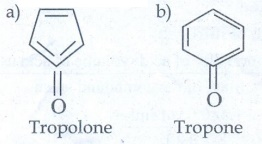iii) Alicyclic

a) Cyclo propane

b) Cyclobutane

iv) Aliphatic open chain

a) CH3 -CH2 - CH2 - CH3 = n - butane

b) CH3 CH2 - CH2 - CH2 - CH3 = n - pentane

2. Write structural formula for the following compounds.

i) Cyclohexa −1, 4 - diene

ii) Ethynyl cyclohexane

Ans :3. Write structural formula for the following compounds (March 19)

i) m - dinitrobenzene

ii) p - dichloro benzene

iii) 1, 3, 5 - Trimethyl benzene

Ans :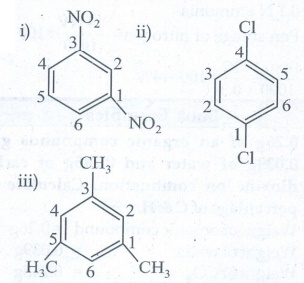4. Write all the possible isomers of molecular formula C4H10O and identify the isomerism found in them.

C4H10O Isomers5. 0.2346g of an organic compound containing C, H & O on combustion gives 0.2754g of H2O and 0.4488 g CO2 Calculate the % composition of C, H & O in the organic compound [C = 52.17, H = 13.04,O = 34.79]

[C = 52.17, H = 13.04, O = 34.79]

Weight of organic substance

(w) = 0.2346g

Weight of water (x) = 0.2754g

Weight of CO2 (y) = 0.4488g

Percentage of carbon = (12/44) × (y/w) × 100

= (12/44) × (0.4488/0.2346) × 100 = 52.17%

Percentage of hydrogen

= (2/18) × (x/w) × 100

= (2/18) × (0.2754/0.2346) × 100 = 13.04%

Percentage of oxygen = [100 - (52.17 + 13.04)]

= 100 – 65.21

= 34.79%

6. 0.16g of an organic compound was heated in a carius tube and H2SO4 acid formed was precipitated with BaCl2 . The mass of BaSO4 was 0.35g. Find the percentage of sulphur.

Weight of organic substance (w) = 0.16g.

Weight of Barium sulphate (x) = 0.35g

Percentage of Sulphur

= (32/233) × (x/w) × 100

= (32/233) × (0.35/0.16) × 100 = 30.04%

7. 0.185g of an organic compound when treated with Cone. HNO3 and silver nitrate gave 0.320g of silver bromide. Calculate the % of bromine in the compound. (Ag = 108, Br = 80)

Weight of organic substance (w) = 0.185g

Weight of silver bromide (x) = 0.320g

Percentage of bromine

= (80/188) × (x / w) × 100

= (80/188) × (0.32/0.185) × 100 = 73.6%

8. 0.40g of an iodo substituted organic compound gave 0.235 g of Agl by carius method. Calculate the percentage of iodine in the compound (Ag = 1081 = 127)

Weight of organic substance

(w) = 0.40g

Weight of silver iodide (x) = 0.235g

Percentage of iodine

= (127/235) × (x/w) × 100

= (127/235) × (0.235/0.40) × 100 = 31.75%

9. 0.33g of an organic compound containing phosphorous gave 0.397 g of Mg2P2O7 by the analysis. Calculate the percentage of P in the compound (MFW of Mg2P2O7 is 222P = 31)

Weight of organic substance (w) = 0.33g

Weight of Mg2P2O7(x) = 0.397g

Percentage of phosphorus

= (62/222) × (x/w) × 100

= (62/222) × (0.397/0.33) × 100 = 33.59%

10. 0.3g of an organic compound on kjeldahl’s analysis gave enough ammonia to just neutralize 30 ml of 0.1 N H2SO4. Calculate the percentage of nitrogen is the compound.

Weight of organic substance(w) = 0.3g

Strength of H2SO4 used (N) = 0.1N

Volume of H2SO4 used (V) = 30 ml

30 ml of 0.1N H2SO4 = 30 ml of 0.1 N ammomia

Percentage of nitrogen = [ 14 NV/1000 ] × 100

[ 14 × 0.1 × 30 ] / [ 1000 × 0.3 ] × 100 = 14%

Study Material, Lecturing Notes, Assignment, Reference, Wiki description explanation, brief detail
11th Chemistry : UNIT 11 : Fundamentals of Organic Chemistry : Brief questions and answers: Fundamentals of Organic Chemistry |